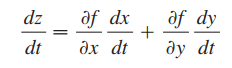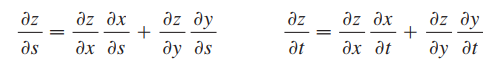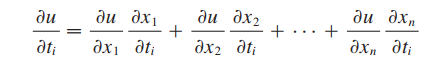Need Help?

Subscribe to Calculus C

###### \${selected_topic_name}
• Notes
• The Chain Rule (Case 1): اذا كان  (z=f (x,y قابلة للاشتقاق على X,Y  ,,  x=g(t) y=h(t )  دوال قابلة للاشتقاق علىt  فيكون :• The Chain Rule (Case 2): اذا كان  (z=f (x,y  قابلة للاشتقاق على X,Y  ,,  x=g(t,s) y=h(t,s)  دوال قابلة للاشتقاق علىt,s  فيكون :• The Chain Rule (General Version):